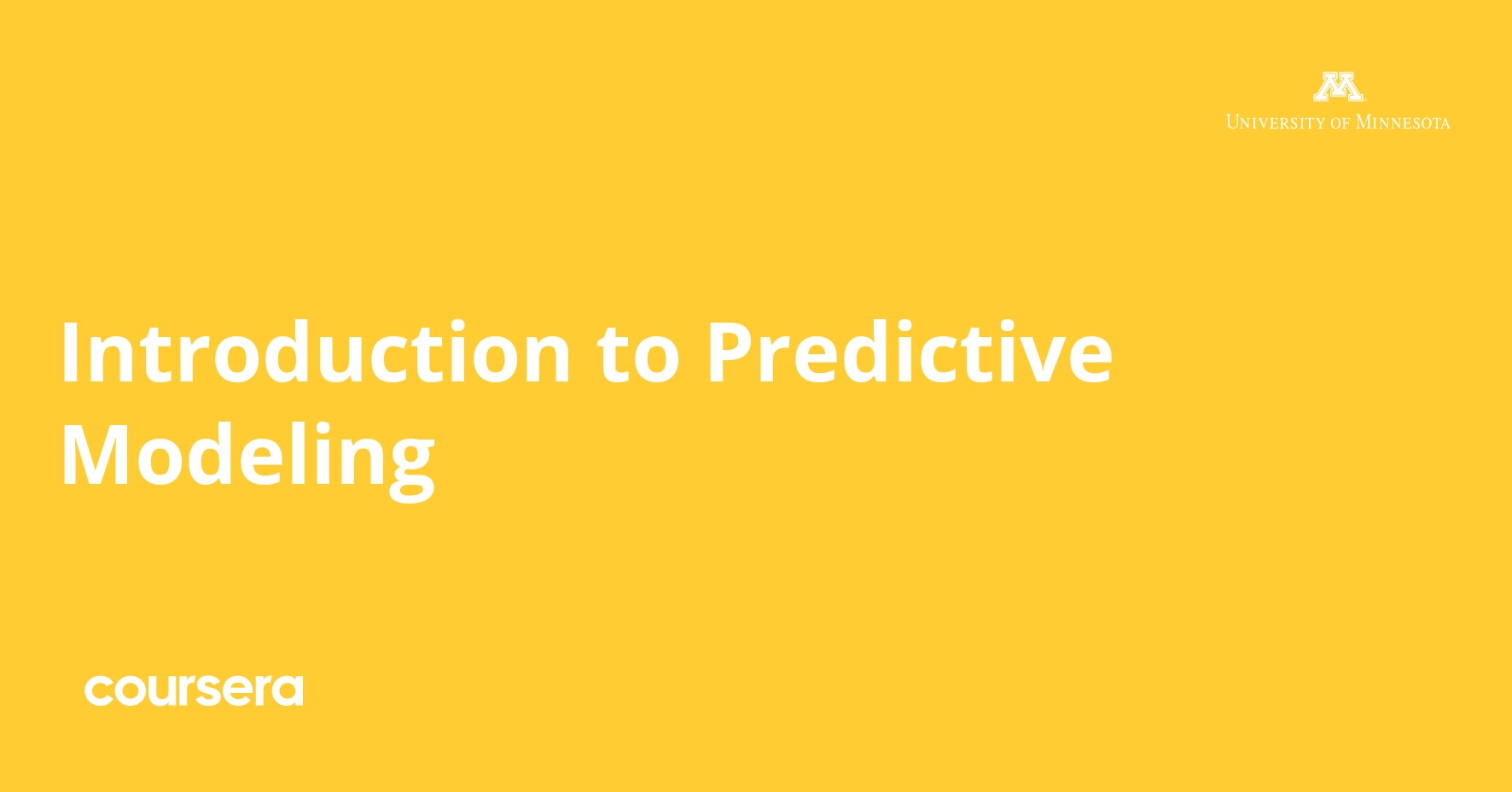# Introduction to Predictive ModelingIntroduction to Predictive Modeling It's a Free Course. Enroll it before the instructor makes it to the paid course.

## By the end of the course, you will be able to learn and Understand:

• How to fit a multiple linear regression model using Excel’s Regression tool and Trend() function
• Making Predictions using the Regression Tool and Trend() function
• How to prepare a dataset for predictive modeling
• Handling Different Types of Variables
• How to use Excel Pivot Table to Explore Column Values
• How to use Excel VLOOKUP to Encode Ordinal Variables
• How to use Excel IF function to Encode Nominal Variables
• How to Forecast using the Moving Averages Model
• Understand the concepts, processes, and applications of predictive modeling.
• Understand the structure of and intuition behind linear regression models.
• Be able to fit simple and multiple linear regression models to data, interpret the results, evaluate the goodness of fit, and use fitted models to make predictions.
• Understand the problem of overfitting and underfitting and be able to conduct simple model selection.
• Understand the concepts, processes, and applications of time series forecasting as a special type of predictive modeling.
• Be able to fit several time-series-forecasting models (e.g., exponential smoothing and Holt-Winter’s method) in Excel, evaluate the goodness of fit, and use fitted models to make forecasts.
• Understand different types of data and how they may be used in predictive models.
• Use Excel to prepare data for predictive modeling, including exploring data patterns, transforming data, and dealing with missing values.
• Understanding the Mechanics of a Regression Model

Course Instructors De Liu & Offered by University of Minnesota from Coursera

Course Duration: Approx. 12 hours